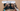# Valid Anagrams - Leet Code Solution

September 10, 2020## Problem Statement

Given two strings s and t , write a function to determine if t is an anagram of s.

Example

``````Input: s = "anagram", t = "nagaram"
Output: true

Input: s = "rat", t = "car"
Output: false``````

Note: You may assume the string contains only lowercase alphabets.

## What is Anagram

First try to understand what an Anagram is. Its NOT about checking order of characters in a string.

• Each character in both strings has equal number of occurrence.

## Solution - 1

A simple solution can be to sort the strings first, then compare.

### Code

``````public boolean isAnagram_sort(String s, String t) {
if (s.length() != t.length()) {
return false;
}
char[] s1 = s.toCharArray();
char[] s2 = t.toCharArray();
Arrays.sort(s1);
Arrays.sort(s2);

return Arrays.equals(s1, s2);
}``````

### Complexity

It is equal to complexity taken by sorting.
Its `O(nlogn)`

## Solution using counting number of characters - HashMap

Another simple solution is that we can use a `HashMap<Character, Integer>`.

• In one pass of first array, we can populate HashMap, which will have count of each character
• In iteration of second array, we can simply decrement count of found characters
• At any time, if the count becomes zero before we decrementing it. Which means, character count do not match.

### Code

``````public boolean isAnagram(String s, String t) {
if (s.length() != t.length()) {
return false;
}

Map<Character, Integer> map = new HashMap<Character, Integer>();
for (int i=0; i<s.length(); i++) {
int count = map.getOrDefault(s.charAt(i), 0);
count ++;

map.put(s.charAt(i), count);
}

for (int i=0; i<t.length(); i++) {
int count = map.getOrDefault(t.charAt(i), 0);
if (count == 0) {
return false;
}

count --;
map.put(t.charAt(i), count);
}

return true;
}``````

### Complexity

Its `O(n)`

## Solution by using array

Since we know that there are only `lowercase characters`. We know the unique number of characters will be `26`.

• We can take an `Integer array` of `count 26`
• We can assume that first index corresponds to `a`, second to `b` and so on.
• In first pass of an array, we can increment count according to location mentioned above
• While iterating second array, we can simply start decrementing count.
• And, at any point if we found the count to be negative. We return false.

### Code

``````public boolean isAnagram_array(String s, String t) {
if (s.length() != t.length()) {
return false;
}

int count[] = new int;
for (int i=0; i<s.length(); i++) {
count[s.charAt(i) - 'a'] ++;
}

for (int i=0; i<t.length(); i++) {
if (count[t.charAt(i) - 'a'] <= 0) {
return false;
}
count[t.charAt(i) - 'a'] --;
}

return true;
}``````

Note that `t.charAt(i) - 'a'` is just to manipulate our indexes.

### Complexity

Its `O(n)`